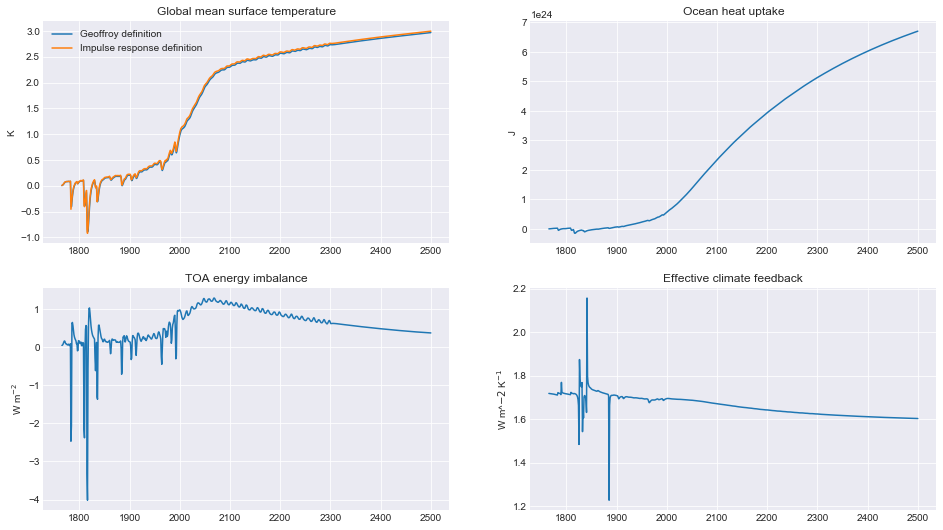# Examples¶

Here are some simple examples of how to run and use the Finite Amplitude Impulse Response (FaIR) model run in the jupyter notebook.

```%matplotlib inline
```
```import fair
fair.__version__
```
```'1.5.1+15.g4289e4f.dirty'
```
```import numpy as np

from matplotlib import pyplot as plt
plt.style.use('seaborn-darkgrid')
plt.rcParams['figure.figsize'] = (16, 9)
```

The “engine” of FaIR is the `fair_scm` function in the `forward` module.

```from fair.forward import fair_scm
```

## CO2 driven run¶

### Basic example¶

Here we show how FaIR can be run with step change CO2 emissions and sinusoidal non-CO2 forcing timeseries. This is a FaIR v1.0-style setup in which CO2 is the only emitted species.

In almost every application of FaIR you will probably want to vary the `emissions` time series going in to `fair_scm`. In CO2-only mode this is a 1D array of CO2 emissions. Setting `useMultigas=False` turns off the emissions from non-CO2 species. To include radiative forcing from non-CO2 forcers, you can specify a constant or a timeseries to the `other_rf` keyword as in the below example.

The output from FaIR is a 3-tuple of `(C,F,T)` arrays. In CO2 mode, both `C` (representing CO2 concentrations in ppm) and `F` (total radiative forcing in W m-2) are 1D arrays. `T` (temperature change since the pre-industrial) is always output as a 1D array.

```# set up emissions and forcing arrays
emissions = np.zeros(250)   # Unit: GtC
emissions[125:] = 10.0
other_rf = np.zeros(emissions.size)
for x in range(0, emissions.size):
other_rf[x] = 0.5 * np.sin(2 * np.pi * (x) / 14.0)

# run the model
C,F,T = fair.forward.fair_scm(
emissions=emissions,
other_rf=other_rf,
useMultigas=False
)

# plot the output
fig = plt.figure()
ax1.plot(range(0, emissions.size), emissions, color='black')
ax1.set_ylabel('Emissions (GtC)')
ax2.plot(range(0, emissions.size), C, color='blue')
ax2.set_ylabel('CO\$_2\$ concentrations (ppm)')
ax3.plot(range(0, emissions.size), F, color='orange')
ax4.plot(range(0, emissions.size), T, color='red')
ax4.set_ylabel('Temperature anomaly (K)');
```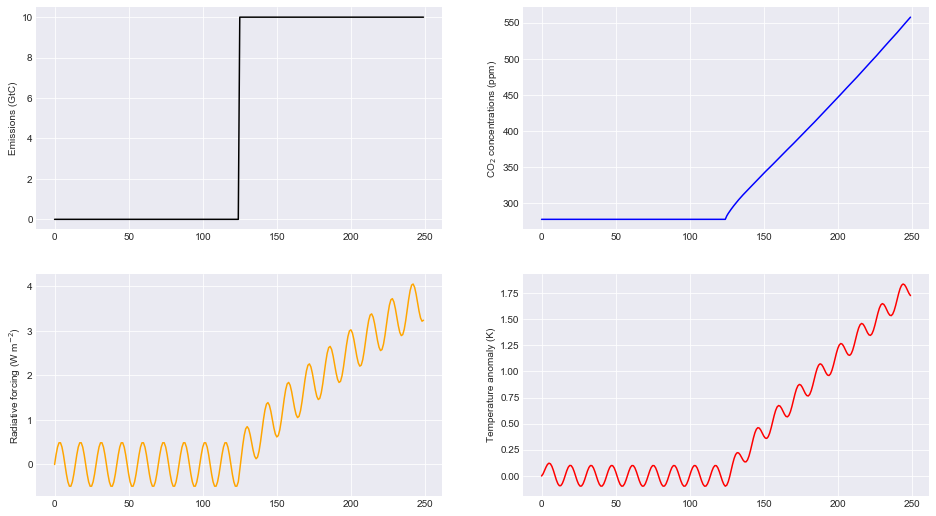### Forcing-only runs¶

If you want to specify a pure forcing and bypass the carbon cycle routine this is also possible by setting `emissions=False`. This time, we will add a linear forcing to the sinusodal forcing above. Note that the CO2 concentrations are not updated from their pre-industrial value.

```# Define a forcing time series
for x in range(0, emissions.size):
other_rf[x] = 0.02*x + 0.5 * np.sin(2 * np.pi * (x) / 14.0)

# run the model with emissions off
_,F,T = fair.forward.fair_scm(
emissions=False,
other_rf=other_rf,
useMultigas=False
)

# plot the output
fig = plt.figure()
ax1.plot(range(0, other_rf.size), F, color='orange')
ax1.plot(range(0, other_rf.size), T, color='red')
ax1.set_ylabel('Temperature anomaly (K)');
```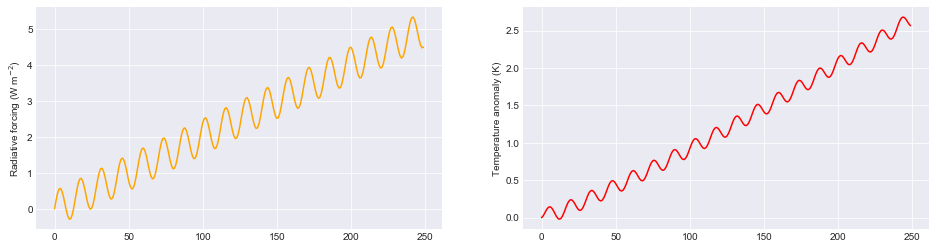### Varying the carbon cycle parameters¶

FaIR is set up to simulate the responses to more complex earth system models. This is achieved by a scaling of a four-box decay model for atmospheric carbon dioxide emissions based on the time-integrated airborne fraction of carbon dioxide. This in turn depends on the efficiency of carbon sinks, which is a function of temperature change and total accumulated carbon uptake. Much of the technical detail is described in Millar et al., (2017).

In the carbon cycle, the important variables are `r0`, `rc` and `rt` which are in turn the pre-industrial sensitivity of carbon sinks, the sensitivity to cumulative carbon dioxide emissions, and sensitivity to temperature change.

This time we will demonstrate with a 10 Gt constant pulse and use a 10-member ensemble.

```# set up emissions and forcing arrays
emissions = np.ones(250) * 10.0   # Unit: GtC
emissions[125:] = 0.0
other_rf = np.zeros(emissions.size)
for x in range(0, emissions.size):
other_rf[x] = 0.5 * np.sin(2 * np.pi * (x) / 14.0)

# create output arrays
nrun = 10
C = np.empty((emissions.size, nrun))
F = np.empty((emissions.size, nrun))
T = np.empty((emissions.size, nrun))

# Generate some random values of carbon cycle parameters
# use a seed for reproducible results
from scipy.stats import norm
r0 = norm.rvs(size=nrun, loc=35, scale=5.0, random_state=42)
rc = norm.rvs(size=nrun, loc=0.019, scale=0.003, random_state=77)
rt = norm.rvs(size=nrun, loc=4.165, scale=0.5, random_state=1729)

# initialise plot
fig = plt.figure()
ax1.plot(range(0, emissions.size), emissions, color='black')
ax1.set_ylabel('Emissions (GtC)')
# run the model and plot outputs
print ("run      r0     rc    rt")
for i in range(nrun):
print ("  %d  %5.3f %5.4f %5.3f" % (i, r0[i], rc[i], rt[i]))
C[:,i],F[:,i],T[:,i] = fair.forward.fair_scm(
emissions=emissions,
other_rf=other_rf,
useMultigas=False,
r0 = r0[i],
rc = rc[i],
rt = rt[i]
)
ax2.plot(range(0, emissions.size), C[:,i], label='run %d' % i)
ax2.set_ylabel('CO\$_2\$ concentrations (ppm)')
ax3.plot(range(0, emissions.size), F[:,i])
ax4.plot(range(0, emissions.size), T[:,i])
ax4.set_ylabel('Temperature anomaly (K)');
ax2.legend();
```
```run      r0     rc    rt
0  37.484 0.0197 3.821
1  34.309 0.0210 3.755
2  38.238 0.0173 4.991
3  42.615 0.0202 3.877
4  33.829 0.0204 4.714
5  33.829 0.0131 4.628
6  42.896 0.0198 3.668
7  38.837 0.0143 3.736
8  32.653 0.0237 4.202
9  37.713 0.0168 4.430
```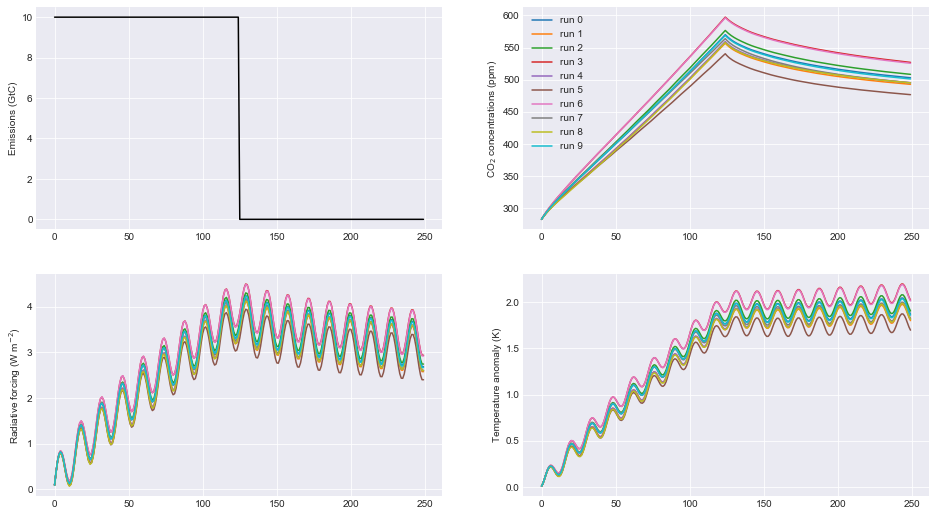### Changing CO2 lifetime and partitioning coefficients¶

The CO2 initial lifetime and partitioning coefficients are quantified by the `tau` and `a` parameters respectively. The rationale follows the four-box model in Myhre et al. (2013), scaled by the impact of land and ocean carbon uptake as described in Millar et al., (2017).

`tau`, in years, is the time constant for each carbon pool and is ordered from slowest carbon pool to fastest, and `a` is the fraction of new CO2 emissions going in to each pool. The first element of `tau` is usually very large and represents the fraction of CO2 emissions that remain in the atmosphere “quasi-permanently”, i.e. removed only on geological time scales, far past the range of times in which FaIR is expected to give useful results (although nobody will stop you using a smaller value as we demonstrate). An error should be thrown if the sum of `a` is not one.

In the second figure it can be seen that these parameter settings are important for the rate of decay of atmospheric CO2 in particular.

```# set up emissions and forcing arrays
emissions = np.ones(250) * 10.0   # Unit: GtC
emissions[125:] = 0.0
other_rf = np.zeros(emissions.size)
for x in range(0, emissions.size):
other_rf[x] = 0.5 * np.sin(2 * np.pi * (x) / 14.0)

# create output arrays
nrun=4
C = np.empty((emissions.size, nrun))
F = np.empty((emissions.size, nrun))
T = np.empty((emissions.size, nrun))

# Play with the carbon boxes
tau2 = np.array([1e6, 400.0, 100.0, 5.0])
a2   = np.ones(4) * 0.25

# Nobody said we had to stick to a four-box model...
tau3 = np.array([1e6, 1000.0, 150.0, 70.0, 15.0, 3.0])
a3   = np.array([0.1, 0.2, 0.2, 0.2, 0.2, 0.1])

# A pathological case where tau0 is much smaller than 1e6
# in this example CO2 behaves more like other GHGs
tau4 = np.array([10., 4., 1., 0.3])
a4   = np.ones(4) * 0.25

# run the model for default values
C[:,0],F[:,0],T[:,0] = fair.forward.fair_scm(
emissions=emissions,
other_rf=other_rf,
useMultigas=False)

# ... and for our alternatives
C[:,1],F[:,1],T[:,1] = fair.forward.fair_scm(
emissions=emissions,
other_rf=other_rf,
useMultigas=False,
tau=tau2,
a=a2)
C[:,2],F[:,2],T[:,2] = fair.forward.fair_scm(
emissions=emissions,
other_rf=other_rf,
useMultigas=False,
tau=tau3,
a=a3)
C[:,3],F[:,3],T[:,3] = fair.forward.fair_scm(
emissions=emissions,
other_rf=other_rf,
useMultigas=False,
tau=tau4,
a=a4)

# plot the output
fig = plt.figure()
ax1.plot(range(0, emissions.size), emissions, color='black')
ax1.set_ylabel('Emissions (GtC)')
handles = ax2.plot(range(0, emissions.size), C)
labels = ['4-box default','4-box alternative','6-box','pathological']
ax2.legend(handles, labels)
ax2.set_ylabel('CO\$_2\$ concentrations (ppm)')
ax3.plot(range(0, emissions.size), F)
ax4.plot(range(0, emissions.size), T)
ax4.set_ylabel('Temperature anomaly (K)');
```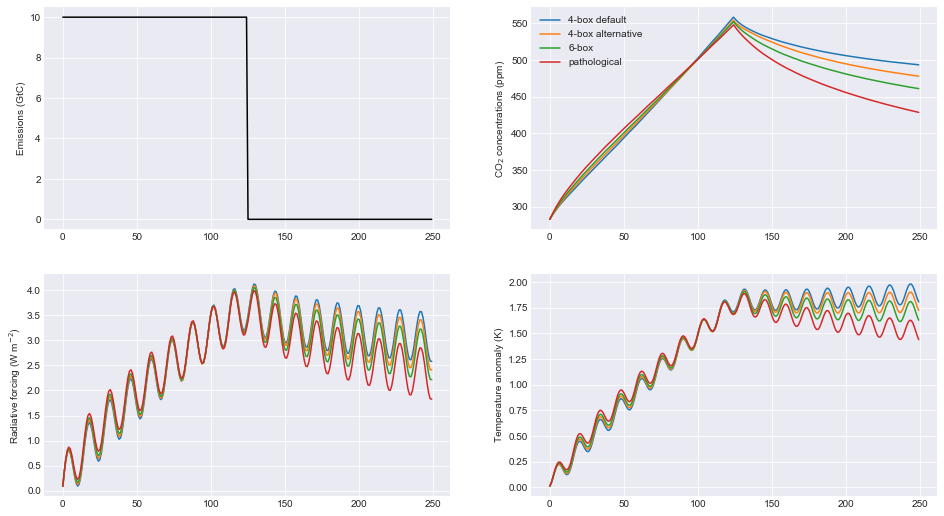### Time-integrated airborne fraction¶

The CO2 time constants, `tau`, are scaled by the 100-year time-integrated airborne fraction for a pulse of CO2. This 100-year time horizon can be changed with the keyword `iirf_h`. The actual time-integrated airborne fraction is a function of `r0`, `rt` and `rc`. In high-emissions scenarios this can get quite high, and if it exceeds `iirf_h` (100 years in the default case) we enter a regime in which there is no solution for the scaling factor. Therefore, a maximum IIRF can be set (`iirf_max`, default value 97 years) which places an upper limit on the time-integrated airborne fraction.

```# set up emissions and forcing arrays
emissions = np.ones(500) * 10.0   # Unit: GtC
other_rf = 0

# create output arrays
nrun=3
C = np.empty((emissions.size, nrun))
F = np.empty((emissions.size, nrun))
T = np.empty((emissions.size, nrun))

# run the model for default values
C[:,0],F[:,0],T[:,0] = fair.forward.fair_scm(
emissions=emissions,
useMultigas=False)
```
```# set iirf time horizon to 60 years. Should get a warning, but it will still let us proceed
C[:,1],F[:,1],T[:,1] = fair.forward.fair_scm(
emissions=emissions,
useMultigas=False,
iirf_h=60)
```
```/nfs/see-fs-02_users/mencsm/FAIR/fair/forward.py:127: RuntimeWarning: iirf_h=60.000000, which is less than iirf_max (97.000000)
% (iirf_h, iirf_max), RuntimeWarning)
```
```# set maximum iirf
C[:,2],F[:,2],T[:,2] = fair.forward.fair_scm(
emissions=emissions,
useMultigas=False,
iirf_h=60,
iirf_max=58)

# plot results
fig = plt.figure()
ax1.plot(range(0, emissions.size), emissions, color='black')
ax1.set_ylabel('Emissions (GtC)')
handles = ax2.plot(range(0, emissions.size), C)
labels = ['iirf_h=100, iirf_max=97','iirf_h=60, iirf_max=97','iirf_h=60, iirf_max=58']
ax2.legend(handles, labels)
ax2.set_ylabel('CO\$_2\$ concentrations (ppm)')
ax3.plot(range(0, emissions.size), F)
ax4.plot(range(0, emissions.size), T)
ax4.set_ylabel('Temperature anomaly (K)');
```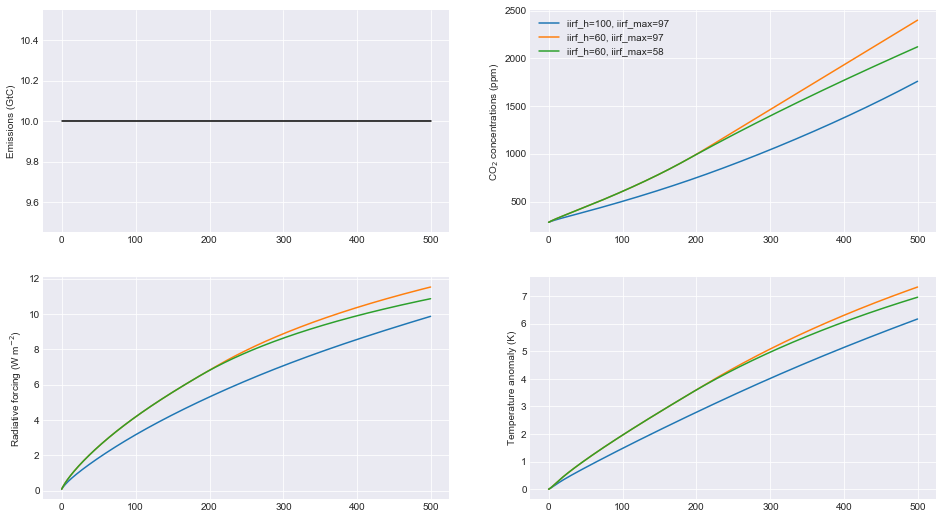### ECS and TCR¶

The equilibrium climate sensitivity (defined as the equilibrium warming for an abrupt doubling of CO2 concentrations) and transient climate response (defined as the temperature change after a CO2 doubling to a 1% per year compound increase in CO2 concentrations - approximately 70 years) are both key uncertainties in climate science. The temperature response in FaIR depends on both. The `tcrecs` parameter, a 2-element array, controls this.

This next example shows the effect of varying the ECS and TCR. (Note that by definition the case ECS=1.0, TCR=1.75 is not possible, but FaIR can handle such cases anyway).

The biggest effect is on the temperature response, but as the temperature feeds back into the carbon cycle, this also affects the CO2 concentrations and the radiative forcing.

```# set up emissions and forcing arrays
emissions = np.zeros(250)
emissions[:125] = 10.0

# create output arrays
nrun=9
C = np.empty((emissions.size, nrun))
F = np.empty((emissions.size, nrun))
T = np.empty((emissions.size, nrun))

# initialise plot
fig = plt.figure()
ax1.plot(range(0, emissions.size), emissions, color='black')
ax1.set_ylabel('Emissions (GtC)')

ecs = np.array([1.0, 2.0, 3.0, 4.0, 5.0, 3.0, 3.0, 3.0, 3.0])
tcr = np.array([1.75, 1.75, 1.75, 1.75, 1.75, 0.75, 1.25, 2.25, 2.75])
colors = ['#800000','#808000','#008000','#008080','#000080', '#000000', '#004000', '#00c000', '#00ff00']

# run the model and plot outputs
for i in range(nrun):
C[:,i],F[:,i],T[:,i] = fair.forward.fair_scm(
emissions=emissions,
useMultigas=False,
tcrecs=np.array([tcr[i], ecs[i]]),
)

ax2.plot(range(0, emissions.size), C[:,i], color=colors[i], label='ECS=%3.1fK, TCR=%4.2fK' % (ecs[i], tcr[i]))
ax2.set_ylabel('CO\$_2\$ concentrations (ppm)')
ax3.plot(range(0, emissions.size), F[:,i], color=colors[i])
ax4.plot(range(0, emissions.size), T[:,i], color=colors[i])
ax4.set_ylabel('Temperature anomaly (K)');
ax2.legend();
```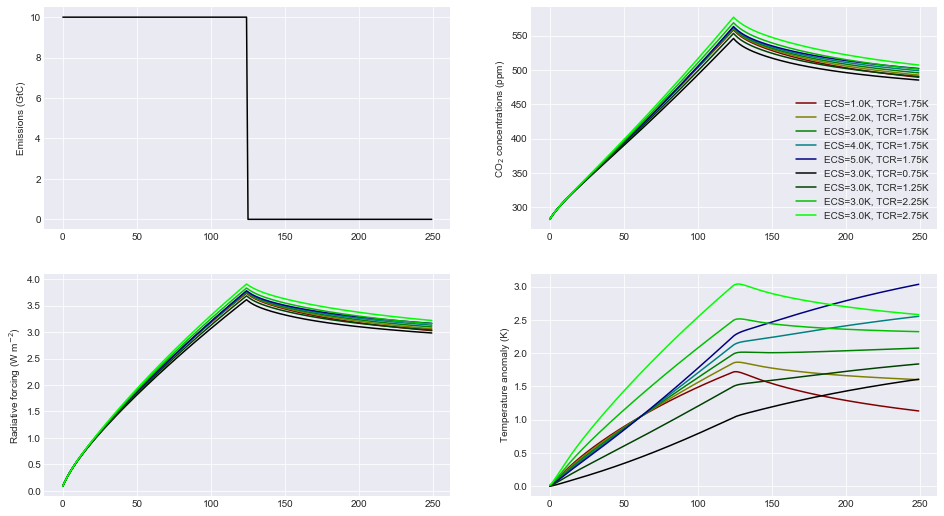Some recent studies (Armour 2017; Gregory and Andrews 2016) suggest that ECS and TCR may not be constant. Fortunately we can investigate this in FaIR by specifying `tcrecs` as a two dimensional `(nt, 2)` array. Notice the effect that a varying ECS/TCR has on the temperature.

```from scipy.stats import lognorm, truncnorm

# generate an ECS time series that roughly follows the AR5 likely range
ecs = lognorm.rvs(0.4, size=250, scale=3, random_state=299)

# define TCR in terms of a realised warming fraction
rwf = truncnorm.rvs(-3, 3, loc=0.6, scale=0.1, size=250, random_state=301)
tcr = rwf*ecs

emissions = np.zeros(250)
emissions[:125] = 10.0

C,F,T = fair.forward.fair_scm(
emissions=emissions,
useMultigas=False,
tcrecs=np.vstack([tcr, ecs]).T,
)

# plot the output
fig = plt.figure()
ax1.plot(range(0, emissions.size), ecs, color='black', label='ECS')
ax1.plot(range(0, emissions.size), tcr, color='black', ls=':', label='TCR')
ax1.legend()
ax1.set_ylabel('ECS/TCR (K)')
ax2.plot(range(0, emissions.size), C, color='blue')
ax2.set_ylabel('CO\$_2\$ concentrations (ppm)')
ax3.plot(range(0, emissions.size), F, color='orange')
ax4.plot(range(0, emissions.size), T, color='red')
ax4.set_ylabel('Temperature anomaly (K)');
```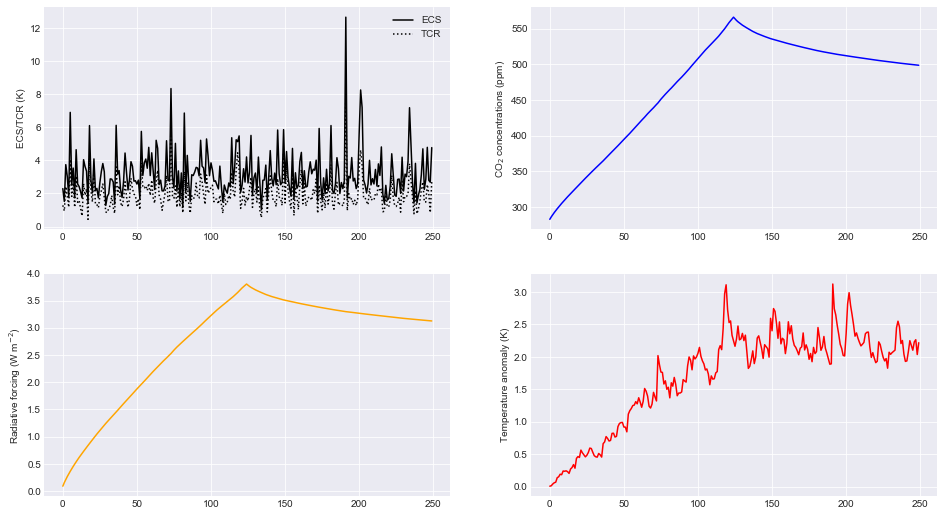The alternative is to specify the values of `q` directly (a 2D array) that go into the temperature calculation, bypassing `tcrecs` completely (setting `tcrecs=None`). It is not known under what circumstances the user may want to do this, but be assured it’s possible!

```# set up emissions and forcing arrays
emissions = np.ones(250) * 10.0
emissions[125:] = 0.0

q = np.ones((250,2))
q[:,0] = 0.2
q[:,1] = 0.6
C,F,T = fair.forward.fair_scm(
emissions=emissions,
useMultigas=False,
tcrecs=None,
q=q,
)
print (C[-1], F[-1], T[-1])
```
```500.5524349046053 3.1476987553820783 2.279051054881363
```

### Temperature time constants¶

The slow and fast response of global mean surface temperature is governed by the two-element array `d`: this parameter determines the rate at which radiative forcing is “realised” as a change in surface temperature.

```# set up emissions and forcing arrays
emissions = np.ones(250) * 10.0   # Unit: GtC
emissions[125:] = 0.0
other_rf = np.zeros(emissions.size)
for x in range(0, emissions.size):
other_rf[x] = 0.5 * np.sin(2 * np.pi * (x) / 14.0)

# create output arrays
nrun=4
C = np.empty((emissions.size, nrun))
F = np.empty((emissions.size, nrun))
T = np.empty((emissions.size, nrun))

# run the model for default values
C[:,0],F[:,0],T[:,0] = fair.forward.fair_scm(
emissions=emissions,
other_rf=other_rf,
useMultigas=False)

# ... and for our alternatives
C[:,1],F[:,1],T[:,1] = fair.forward.fair_scm(
emissions=emissions,
other_rf=other_rf,
useMultigas=False,
d=np.array([1000.0, 18.0]))
C[:,2],F[:,2],T[:,2] = fair.forward.fair_scm(
emissions=emissions,
other_rf=other_rf,
useMultigas=False,
d=np.array([239.0, 1.0]))
C[:,3],F[:,3],T[:,3] = fair.forward.fair_scm(
emissions=emissions,
other_rf=other_rf,
useMultigas=False,
d=np.array([60., 4.1]))

# plot the output
fig = plt.figure()
ax1.plot(range(0, emissions.size), emissions, color='black')
ax1.set_ylabel('Emissions (GtC)')
handles = ax2.plot(range(0, emissions.size), C)
labels = ['default','slow repsonse','quick mixed layer response','quick deep ocean response']
ax2.legend(handles, labels)
ax2.set_ylabel('CO\$_2\$ concentrations (ppm)')
ax3.plot(range(0, emissions.size), F)
ax4.plot(range(0, emissions.size), T)
ax4.set_ylabel('Temperature anomaly (K)');
```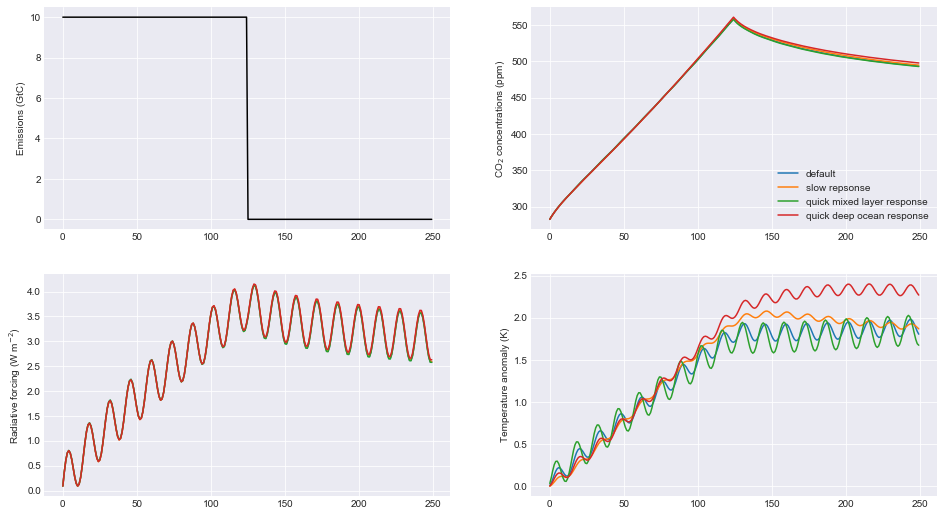## Multi-species mode¶

More interesting scenarios can be created with the full suite of forcing agents. The key changes from CO2-only mode that should be remembered are:

• This time the emissions dataset is a (nt, 40) array of inputs

• `useMultigas` should be set to `True`, or omitted (this is the default option)

• The concentration and forcing outputs are themselves 2-dimensional arrays of size (nt, 31) and (nt, 13) respectively

• More input options to `fair_scm` become available.

The basic call to fair_scm remains the same:

```(C,F,T) = fair_scm(emissions=emissions, **kwargs)
```

### Emissions¶

In multi-species model, emissions are input as an `(nt, 40)` emissions array. The index order and units of the columns are as follows:

Index

Species

Units

0

Year

year

1

CO2-fossil

GtC/yr

2

CO2-landuse

GtC/yr

3

CH4

Mt/yr

4

N2O

MtN2/yr

5

SOx

MtS/yr

6

CO

Mt/yr

7

NMVOC

Mt/yr

8

NOx

MtN/yr

9

BC

Mt/yr

10

OC

Mt/yr

11

NH3

Mt/yr

12

CF4

kt/yr

13

C2F6

kt/yr

14

C6F14

kt/yr

15

HFC23

kt/yr

16

HFC32

kt/yr

17

HFC43-10

kt/yr

18

HFC125

kt/yr

19

HFC134a

kt/yr

20

HFC143a

kt/yr

21

HFC227ea

kt/yr

22

HFC245fa

kt/yr

23

SF6

kt/yr

24

CFC11

kt/yr

25

CFC12

kt/yr

26

CFC113

kt/yr

27

CFC114

kt/yr

28

CFC115

kt/yr

29

CCl4

kt/yr

30

Methyl chloroform

kt/yr

31

HCFC22

kt/yr

32

HCFC141b

kt/yr

33

HCFC142b

kt/yr

34

Halon 1211

kt/yr

35

Halon 1202

kt/yr

36

Halon 1301

kt/yr

37

Halon 2401

kt/yr

38

CH3Br

kt/yr

39

CH3Cl

kt/yr

The index order of the columns follows that of the RCP datasets at http://www.pik-potsdam.de/~mmalte/rcps/.

### GHG Concentrations¶

Multi-species FaIR tracks the atmospheric concentrations of 31 GHG species; `C` is returned as a `(nt, 31)` array. The colums are indexed as follows:

Index

Species

Units

0

CO2

ppm

1

CH4

ppb

2

N2O

ppb

3

CF4

ppt

4

C2F6

ppt

5

C6F14

ppt

6

HFC23

ppt

7

HFC32

ppt

8

HFC43-10

ppt

9

HFC125

ppt

10

HFC134a

ppt

11

HFC143a

ppt

12

HFC227ea

ppt

13

HFC245fa

ppt

14

SF6

ppt

15

CFC11

ppt

16

CFC12

ppt

17

CFC113

ppt

18

CFC114

ppt

19

CFC115

ppt

20

CCl4

ppt

21

Methyl chloroform

ppt

22

HCFC22

ppt

23

HCFC141b

ppt

24

HCFC142b

ppt

25

Halon 1211

ppt

26

Halon 1202

ppt

27

Halon 1301

ppt

28

Halon 2401

ppt

29

CH3Br

ppt

30

CH3Cl

ppt

Finally, a `(nt, 13)` array `F` of effective radiative forcing is returned (all units W m-2):

Index

Species

0

CO2

1

CH4

2

N2O

3

All other well-mixed GHGs

4

Tropospheric O3

5

Stratospheric O3

6

Stratospheric water vapour from CH4 oxidation

7

Contrails

8

Aerosols

9

Black carbon on snow

10

Land use change

11

Volcanic

12

Solar

With the exception of volcanic and solar, all forcing outputs are calculated from the input emissions.

## A multi-gas example¶

This sets up a multi-gas emissions array and serves to demonstrate some of the options that can be specified in `fair_scm` for multi-gas runs (most are changed from the default and some are non-sensical but shown for illustration). Note this is a completely hypothetical scenario!

```from scipy.stats import gamma
emissions = np.zeros((250,40))

# remember column zero is the years
emissions[:,0] = np.arange(1850,2100)

# add some CO2 fossil and land use, GtC/yr
emissions[:,1] = 10.
emissions[:,2] = 1.

# some methane and nitrous oxide in this example, Mt/yr
emissions[:,3] = 300.
emissions[:,4] = 19.

# aerosol and ozone precursors, Mt/yr
emissions[:,5] = 0.1*np.arange(250)   # SOx
emissions[:,6] = 500.*np.log(1+np.arange(250))  # CO
emissions[:,7] = 100.+100.*np.cos(np.arange(250))  # NMVOC
emissions[:,8] = 40.*norm.rvs(loc=1, scale=0.1, size=250, random_state=9)   # NOx
emissions[:,9] = 6.    # BC
emissions[:,10] = 30.  # OC
emissions[:,11] = 35.  # NH3

# throw in some CFCs
emissions[:,24] = 1000. # CFC11
# and leave all other emissions as zero.

# Volcanic and solar forcing are provided externally. Let's invent some
solar = 0.1 * np.sin(2 * np.pi * np.arange(250) / 11.5)
volcanic = -gamma.rvs(0.2, size=250, random_state=100)

# efficacies are the temperature change for each forcing agent compared to CO2
# in our runs we usually set the efficacy of BC on snow to 3, following Bond et
# al (2013)
eff = np.ones(13)
eff = 3.0

# b_aero: ERFari for each SLCF species (indices 5 to 11)
# b_tro3: tropospheric ozone coeffs for CH4, CO, NMVOC, NOx
C,F,T = fair_scm(emissions=emissions,
natural=np.zeros((250,2)), # natural emissions of CH4 and N2O
aviNOx_frac=0.05, # proportion of NOx emissions from aviation
fossilCH4_frac=0.25, # proportion of anthro CH4 emis from fossil fuels
oxCH4_frac=0.61, # proportion of fossil CH4 eventually oxidised to CO2
stwv_from_ch4=0.1, # proportion of CH4 ERF contributing to strat H2O
ghg_forcing='Etminan',  # etminan or myhre
useStevenson=False, # Stevenson or regression based trop. O3 forcing?
b_aero = np.array([-35,0,-5,-6,450,-40,-10])*1e-4,
b_tro3 = np.array([3., 1., 8., 99.])*1e-4,
aerosol_forcing = 'aerocom+ghan',  # aerocom, aerocom+ghan or stevens
F_solar = solar,
F_volcanic = volcanic,
efficacy = eff
)

# Plot the forcing from each component
fig = plt.figure()
label = ['CO2','CH4','N2O','Other GHG','Trop O3','Strat O3','Strat H2O','Contrails','Aerosols',
'BC on snow', 'Land use', 'Volcanic', 'Solar']
for i in range(13):
ax.plot(np.arange(1850,2100), F[:,i])
ax.text(0.95,0.95,label[i],transform=ax.transAxes,va='top', ha='right')
# plot temperature change
ax.plot(np.arange(1850,2100),T)
ax.text(0.95, 0, 'Temperature change', transform=ax.transAxes, va='bottom', ha='right')
```
```Text(0.95, 0, 'Temperature change')
```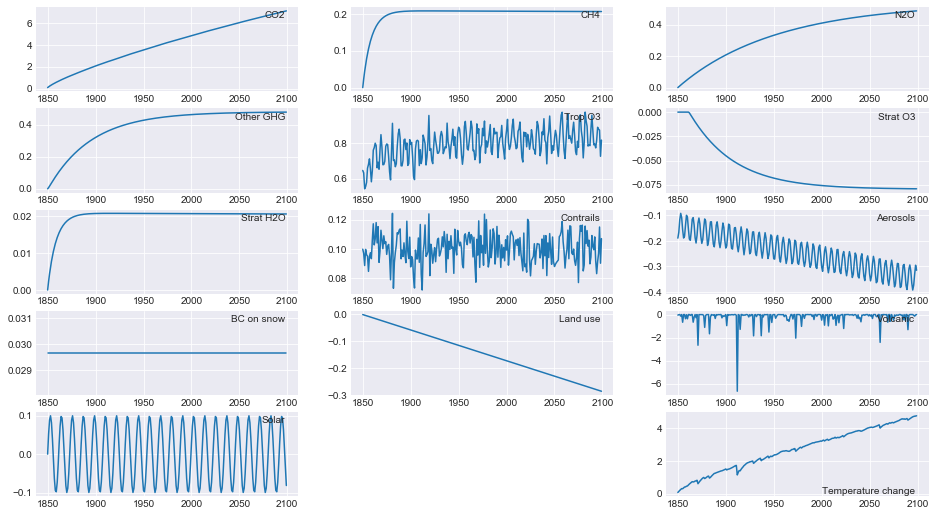## RCP scenarios¶

Creating a 40-column emissions input table may seem a lot of work. FaIR comes with tools to make your life easier!

We can run FaIR with the CO2 emissions and non-CO2 forcing from the four representative concentration pathway scenarios. These can be imported from the `RCPs` module and have inbuilt `Forcing` and `Emissions` classes. There is also a tool for converting MAGICC6 *.SCEN files into FaIR input (in `fair/tools/magicc`).

Here we show the FaIR implementation of the RCP scenarios.

```# Get RCP modules
from fair.RCPs import rcp26, rcp45, rcp60, rcp85

# Basic RCP runs
C26, F26, T26 = fair.forward.fair_scm(emissions=rcp26.Emissions.emissions)
C45, F45, T45 = fair.forward.fair_scm(emissions=rcp45.Emissions.emissions)
C60, F60, T60 = fair.forward.fair_scm(emissions=rcp60.Emissions.emissions)
C85, F85, T85 = fair.forward.fair_scm(emissions=rcp85.Emissions.emissions)

fig = plt.figure()

ax1.plot(rcp26.Emissions.year, rcp26.Emissions.co2_fossil, color='green', label='RCP2.6')
# just show CO2 conc.
ax2.plot(rcp26.Emissions.year, C26[:, 0], color='green')
# sum over axis 1 to get total ERF
ax3.plot(rcp26.Emissions.year, np.sum(F26, axis=1), color='green')
ax4.plot(rcp26.Emissions.year, T26, color='green')

ax1.plot(rcp45.Emissions.year, rcp45.Emissions.co2_fossil, color='blue', label='RCP4.5')
ax2.plot(rcp45.Emissions.year, C45[:, 0], color='blue')
ax3.plot(rcp45.Emissions.year, np.sum(F45, axis=1), color='blue')
ax4.plot(rcp45.Emissions.year, T45, color='blue')

ax1.plot(rcp60.Emissions.year, rcp60.Emissions.co2_fossil, color='red', label='RCP6')
ax2.plot(rcp60.Emissions.year, C60[:, 0], color='red')
ax3.plot(rcp60.Emissions.year, np.sum(F60, axis=1), color='red')
ax4.plot(rcp60.Emissions.year, T60, color='red')

ax1.plot(rcp85.Emissions.year, rcp85.Emissions.co2_fossil, color='black', label='RCP8.5')
ax2.plot(rcp85.Emissions.year, C85[:, 0], color='black')
ax3.plot(rcp85.Emissions.year, np.sum(F85, axis=1), color='black')
ax4.plot(rcp85.Emissions.year, T85, color='black')

ax1.set_ylabel('Fossil CO\$_2\$ Emissions (GtC)')
ax1.legend()
ax2.set_ylabel('CO\$_2\$ concentrations (ppm)')
ax4.set_ylabel('Temperature anomaly (K)');
```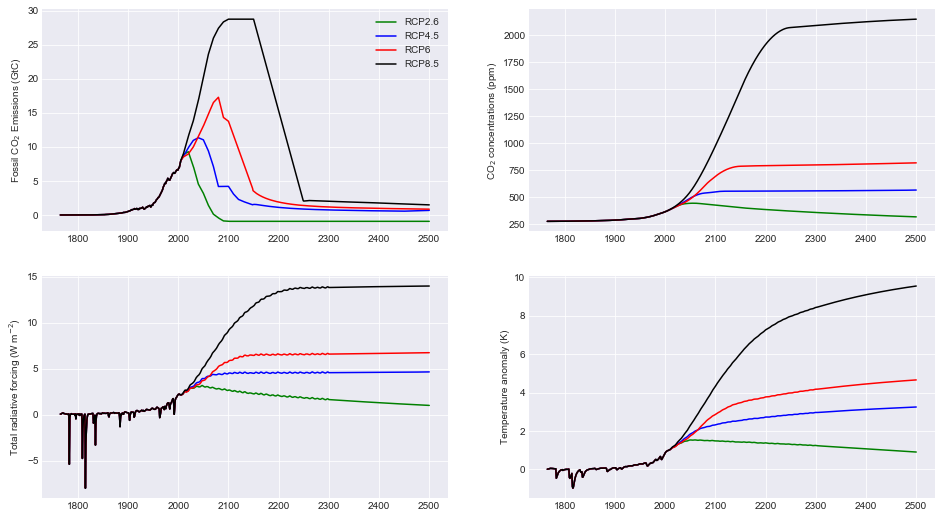## Concentrations of well-mixed greenhouse gases¶

In this example we also show how to group minor gases into CFC12 and HFC134a equivalent concentrations. Refer to table above for gas indices.

```fig = plt.figure()

ax1.plot(rcp26.Emissions.year, C26[:,1], color='green', label='RCP3PD')
ax1.plot(rcp45.Emissions.year, C45[:,1], color='blue', label='RCP4.5')
ax1.plot(rcp60.Emissions.year, C60[:,1], color='red', label='RCP6')
ax1.plot(rcp85.Emissions.year, C85[:,1], color='black', label='RCP8.5')
ax1.set_title("Methane concentrations, ppb")

ax2.plot(rcp26.Emissions.year, C26[:,2], color='green', label='RCP3PD')
ax2.plot(rcp45.Emissions.year, C45[:,2], color='blue', label='RCP4.5')
ax2.plot(rcp60.Emissions.year, C60[:,2], color='red', label='RCP6')
ax2.plot(rcp85.Emissions.year, C85[:,2], color='black', label='RCP8.5')
ax2.set_title("Nitrous oxide concentrations, ppb")

# Weight H and F gases by radiative efficiency

# indices 3:15 are HFCs and PFCs

# indices 15:31 are ozone depleters

ax3.plot(rcp26.Emissions.year, C26_hfc134a_eq, color='green', label='RCP2.6')
ax3.plot(rcp45.Emissions.year, C45_hfc134a_eq, color='blue', label='RCP4.5')
ax3.plot(rcp60.Emissions.year, C60_hfc134a_eq, color='red', label='RCP6')
ax3.plot(rcp85.Emissions.year, C85_hfc134a_eq, color='black', label='RCP8.5')
ax3.set_title("HFC134a equivalent concentrations, ppt")

ax4.plot(rcp26.Emissions.year, C26_cfc12_eq, color='green', label='RCP2.6')
ax4.plot(rcp45.Emissions.year, C45_cfc12_eq, color='blue', label='RCP4.5')
ax4.plot(rcp60.Emissions.year, C60_cfc12_eq, color='red', label='RCP6')
ax4.plot(rcp85.Emissions.year, C85_cfc12_eq, color='black', label='RCP8.5')
ax4.set_title("CFC12 equivalent concentrations, ppt")
ax1.legend();
```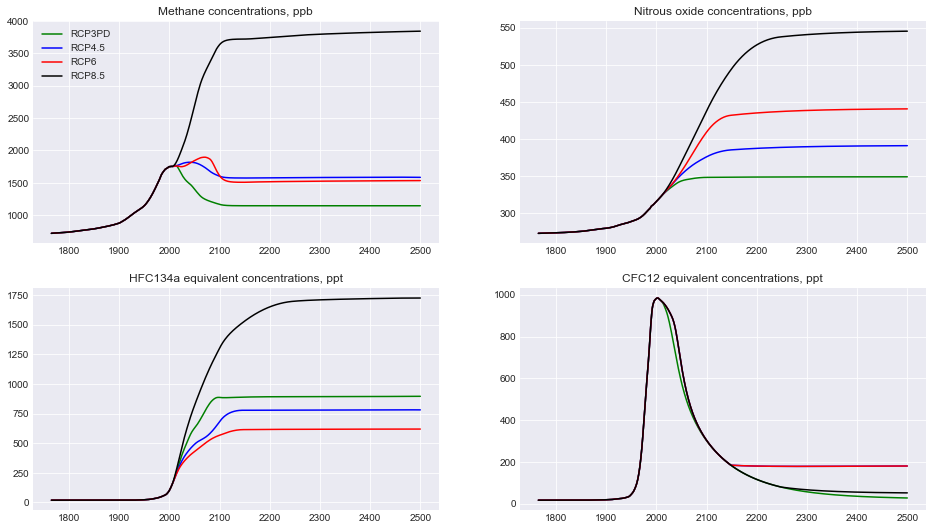Here we show some of the more interesting examples for the effective radiative forcing time series coming out of FaIR.

```fig = plt.figure()

ax1.plot(rcp26.Emissions.year, F26[:,4], color='green', label='RCP2.6')
ax1.plot(rcp45.Emissions.year, F45[:,4], color='blue', label='RCP4.5')
ax1.plot(rcp60.Emissions.year, F60[:,4], color='red', label='RCP6')
ax1.plot(rcp85.Emissions.year, F85[:,4], color='black', label='RCP8.5')
ax1.set_title("Tropospheric ozone forcing, W m\$^{-2}\$")

ax2.plot(rcp26.Emissions.year, F26[:,5], color='green', label='RCP2.6')
ax2.plot(rcp45.Emissions.year, F45[:,5], color='blue', label='RCP4.5')
ax2.plot(rcp60.Emissions.year, F60[:,5], color='red', label='RCP6')
ax2.plot(rcp85.Emissions.year, F85[:,5], color='black', label='RCP8.5')
ax2.set_title("Stratospheric ozone forcing, W m\$^{-2}\$")

ax3.plot(rcp26.Emissions.year, F26[:,8], color='green', label='RCP2.6')
ax3.plot(rcp45.Emissions.year, F45[:,8], color='blue', label='RCP4.5')
ax3.plot(rcp60.Emissions.year, F60[:,8], color='red', label='RCP6')
ax3.plot(rcp85.Emissions.year, F85[:,8], color='black', label='RCP8.5')
ax3.set_title("Aerosol forcing, W m\$^{-2}\$")

ax4.plot(rcp26.Emissions.year, F26[:,10], color='green', label='RCP2.6')
ax4.plot(rcp45.Emissions.year, F45[:,10], color='blue', label='RCP4.5')
ax4.plot(rcp60.Emissions.year, F60[:,10], color='red', label='RCP6')
ax4.plot(rcp85.Emissions.year, F85[:,10], color='black', label='RCP8.5')
ax4.set_title("Land use forcing, W m\$^{-2}\$")
ax1.legend();
```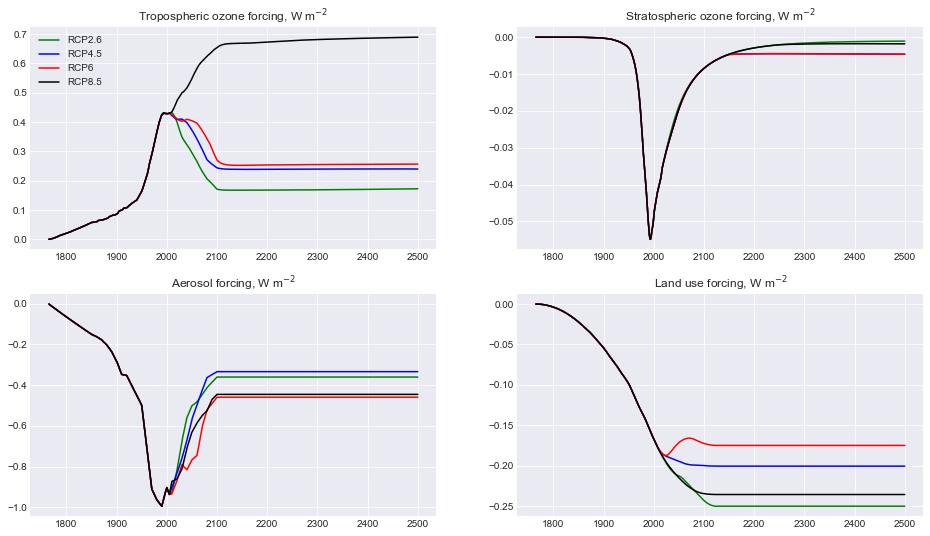## Running in concentration-driven mode¶

It is possible to drive FaIR with concentrations rather than emissions. Set the `emissions_driven` keyword to `False` and specify the concentrations with `C`. The function still returns the `C, F, T` tuple; comparison of the input concentrations with the output concentrations can be performed as a sense check to verify the code is not changing anything.

### CO2 only¶

```# Produce a base emissions-driven RCP4.5 run
C1,F1,T1 = fair.forward.fair_scm(
emissions_driven=True,
emissions=fair.RCPs.rcp45.Emissions.co2,
useMultigas=False)

# Produce a concentration driven RCP4.5 run
C2,F2,T2 = fair.forward.fair_scm(
emissions_driven=False,
C=fair.RCPs.rcp45.Concentrations.co2,
useMultigas=False)

fig = plt.figure()

ax1.plot(rcp45.Emissions.year, F1, color='blue', label='Emissions driven')
ax1.plot(rcp45.Emissions.year, F2, color='red', label='Concentrations driven')

ax2.plot(rcp45.Emissions.year, T1, color='blue', label='Emissions driven')
ax2.plot(rcp45.Emissions.year, T2, color='red', label='Concentrations driven')
ax2.set_title("RCP4.5 temperature anomaly, K")
ax1.legend();
```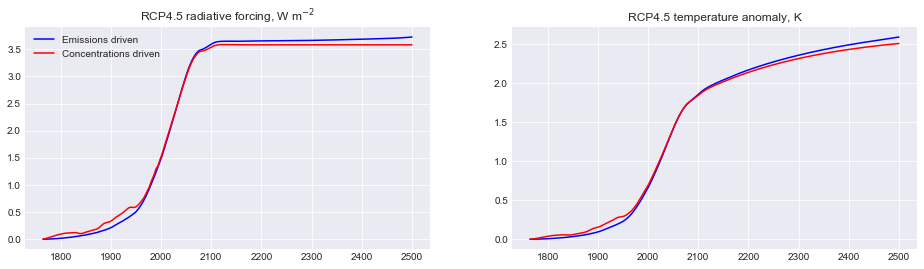### Diagnosing CO2 emissions¶

For carbon budget calculations it is often useful to calculate (cumulative) CO2 emissions for a given concentration of CO2. The `fair.inverse` module provides the opportunity to do this. Currently `fair.inverse` only runs in CO2 mode.

```from fair.inverse import inverse_fair_scm
```

Many of the options are the same as for the `forward` model, and provide reasonable sensible defaults if not specified. The classic example of a 1% per year CO2 increase will be demonstrated.

```nt = 140 # years
C  = 278. * 1.01**np.arange(nt) # compound 1% increase in CO2 concentrations
```
```# run with default carbon cycle parameters
E1, F1, T1 = inverse_fair_scm(C=C)

# show the effect of turning off the temperature feedback
E2, F2, T2 = inverse_fair_scm(C=C, rt=0)

fig = plt.figure()
ax1.plot(range(nt), E1, color='blue', label='Temperature feedback')
ax1.plot(range(nt), E2, color='red', label='No temperature feedback')
ax1.set_title("Diagnosed emissions from 1% CO2 run")
ax1.set_xlabel('year')
ax1.set_ylabel('GtC/yr')
ax2.plot(np.cumsum(E1), T1, color='blue')
ax2.plot(np.cumsum(E2), T1, color='red')
ax2.set_title("Transient climate response to cumulative CO2 emissions (TCRE)")
ax2.set_xlabel("Cumulative emissions, GtC")
ax2.set_ylabel("Temperature anomaly, K")
ax1.legend();
```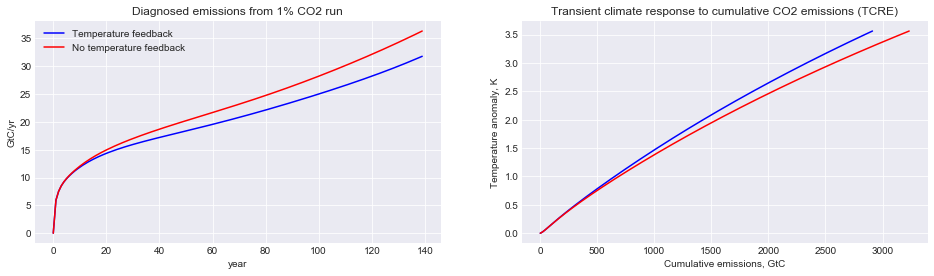### Multi-gas¶

In multi-gas concentrations driven mode, all non-WMGHG forcing that is normally calculated from emissions (tropospheric ozone, aviation contrails, aerosols, black carbon on snow and land use) is provided externally. The default values for each are zero. WMGHG forcing that is calculated from concentrations or forcing (stratospheric ozone and stratospheric water vapour from methane) is not affected.

```# Produce a base emissions-driven RCP4.5 run
C1,F1,T1 = fair.forward.fair_scm(
emissions_driven=True,
emissions=fair.RCPs.rcp45.Emissions.emissions,
useMultigas=True)

# Produce a concentration driven RCP4.5 run
# use Meinshausen's external forcing here
C2,F2,T2 = fair.forward.fair_scm(
emissions_driven=False,
C=fair.RCPs.rcp45.Concentrations.gases,
F_tropO3 = rcp45.Forcing.tropo3,
F_aerosol = rcp45.Forcing.aero+rcp45.Forcing.cloud,
F_bcsnow = rcp45.Forcing.bcsnow,
useMultigas=True)

fig = plt.figure()

ax1.plot(rcp45.Emissions.year, np.sum(F1,axis=1), color='blue', label='Emissions driven')
ax1.plot(rcp45.Emissions.year, np.sum(F2,axis=1), color='red', label='Concentrations driven')

ax2.plot(rcp45.Emissions.year, T1, color='blue', label='Emissions driven')
ax2.plot(rcp45.Emissions.year, T2, color='red', label='Concentrations driven')
ax2.set_title("RCP4.5 temperature anomaly, K")
ax1.legend();
```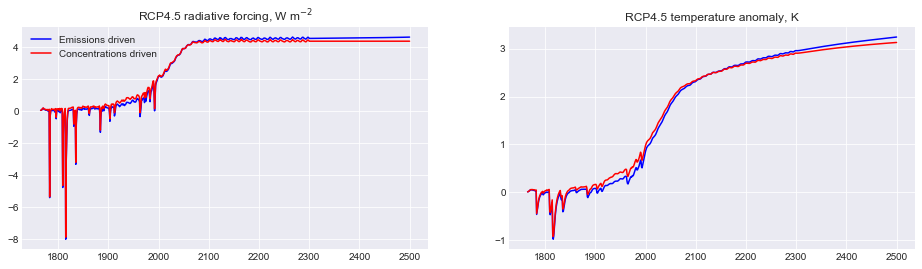## Natural emissions and GHG lifetimes¶

In order to balance historical concentrations of methane and nitrous oxide, we assume a time-varying profile of natural emissions. This can be varied with the `natural` keyword (a `(nt, 2)` array of methane and nitrous oxide emissions). Additionally, the default greenhouse gas decay constants can be modified with the `lifetimes` keyword (shape `(31,)`).

It can clearly be seen that natural emissions are important in maintaining historical concentrations.

```# Change default lifetimes of CH4 and N2O
lt = 12.6
lt = 131.

# what are the defaults?

# How long are the RCPs?
nt = len(rcp45.Emissions.year)

# Run FaIR under RCP4.5 with no natural emissions
C1,F1,T1 = fair_scm(emissions=rcp45.Emissions.emissions,
natural=np.zeros((nt,2))
)

# Run FaIR under RCP4.5 with modified lifetimes
C2,F2,T2 = fair_scm(emissions=rcp45.Emissions.emissions,
)

fig = plt.figure()

ax1.plot(rcp45.Emissions.year, C45[:,1], color='blue', label='RCP4.5 default')
ax1.plot(rcp45.Emissions.year, C1[:,1], color='blue', ls=':', label='RCP4.5 no natural')
ax1.plot(rcp45.Emissions.year, C2[:,1], color='blue', ls='--', label='RCP4.5 modified lifetime')
ax1.set_title("Methane concentrations, ppb")
ax2.plot(rcp45.Emissions.year, C45[:,2], color='blue', label='RCP4.5')
ax2.plot(rcp45.Emissions.year, C1[:,2], color='blue', ls=':', label='RCP4.5 no natural')
ax2.plot(rcp45.Emissions.year, C2[:,2], color='blue', ls='--', label='RCP4.5 modified lifetime')
ax2.set_title("Nitrous oxide concentrations, ppb")
ax1.legend();
```
```9.3 121.0
```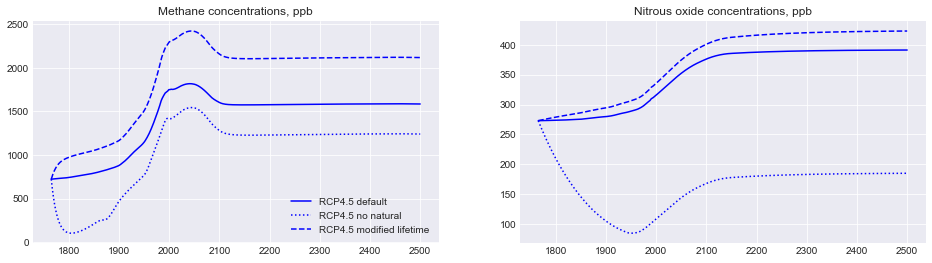## Ensemble generation¶

An advantage of FaIR is that it is very quick to run (much less than a second on an average machine). Therefore it can be used to generate probabilistic future ensembles. We’ll show a 100-member ensemble.

FaIR comes with a built-in ensemble generator that takes into account the fact that ECS and TCR are highly correlated (at least in CMIP5 models). A joint-lognormal or joint-normal distribution of ECS and TCR can be generated, and optionally the correlation can be switched off. See the documentation for `fair.tools.ensemble.ecstcr_generate` for details.

This example also introduces the `scale` and `F2x` keywords. `scale` (a 13 element array) governs the forcing scaling factor of each of the 13 categories of forcing, whereas `F2x` determines the ERF from a doubling of CO2.

```from scipy import stats
from fair.tools.ensemble import tcrecs_generate

# generate 100 TCR and ECS pairs, using a lognormal distribution informed by CMIP5 models
tcrecs = tcrecs_generate('cmip5', n=100, dist='lognorm', correlated=True, seed=38571)

# generate some forcing scale factors with SD of 10% of the best estimate
F_scale = stats.norm.rvs(size=(100,13), loc=1, scale=0.1, random_state=40000)
F2x = 3.71 * F_scale[:,0]
F_scale[:,0] = 1.0  # set CO2 forcing scaling with F2x above

# generate ensemble for carbon cycle parameters
r0 = stats.norm.rvs(size=100, loc=35, scale=3.5, random_state=41000)
rc = stats.norm.rvs(size=100, loc=0.019, scale=0.0019, random_state=42000)
rt = stats.norm.rvs(size=100, loc=4.165, scale=0.4165, random_state=45000)

T = np.zeros((nt,100))

# notice that we
for i in range(100):
_, _, T[:,i] = fair_scm(emissions=rcp85.Emissions.emissions,
r0 = r0[i],
rc = rc[i],
rt = rt[i],
tcrecs = tcrecs[i,:],
scale = F_scale[i,:],
F2x = F2x[i]
)
```
```fig = plt.figure()
ax1.plot(rcp85.Emissions.year, T);
```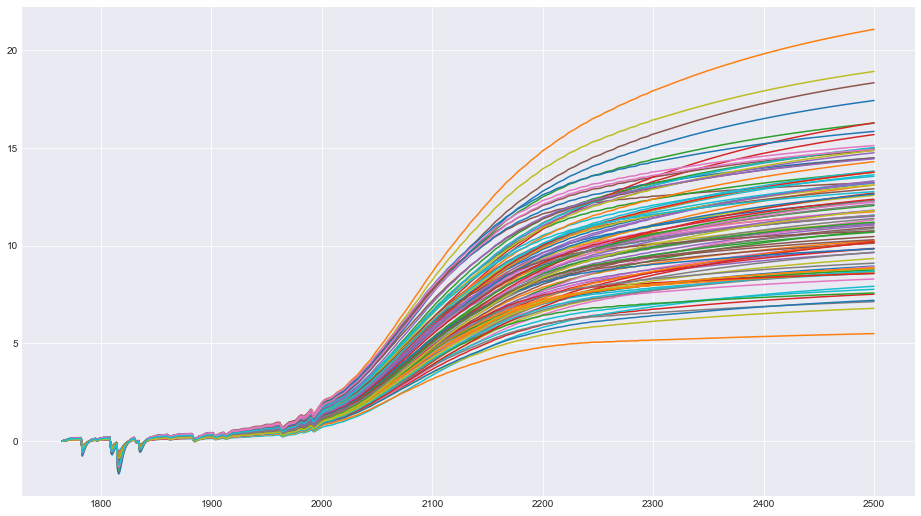The resulting projections show a large spread. Some of these ensemble members are unrealistic, ranging from around 0.4 to 2.0 K temperature change in the present day, whereas we know in reality it is more like 0.95 (plus or minus 0.2). Therefore we can constrain this ensemble to observations.

```from fair.tools.constrain import hist_temp

# Cowtan & Way in-filled dataset of global temperatures
constrained = np.zeros(100, dtype=bool)
for i in range(100):
# we use observed trend from 1880 to 2016
constrained[i],_,_,_,_ = hist_temp(
CW[30:,1], T[1880-1765:2017-1765,i], CW[30:,0])
```
```# How many ensemble members passed the constraint?
print (np.sum(constrained))
```
```31
```
```# What does this do to the ensemble?
fig = plt.figure()
ax1.plot(rcp85.Emissions.year, T[:,constrained]);
```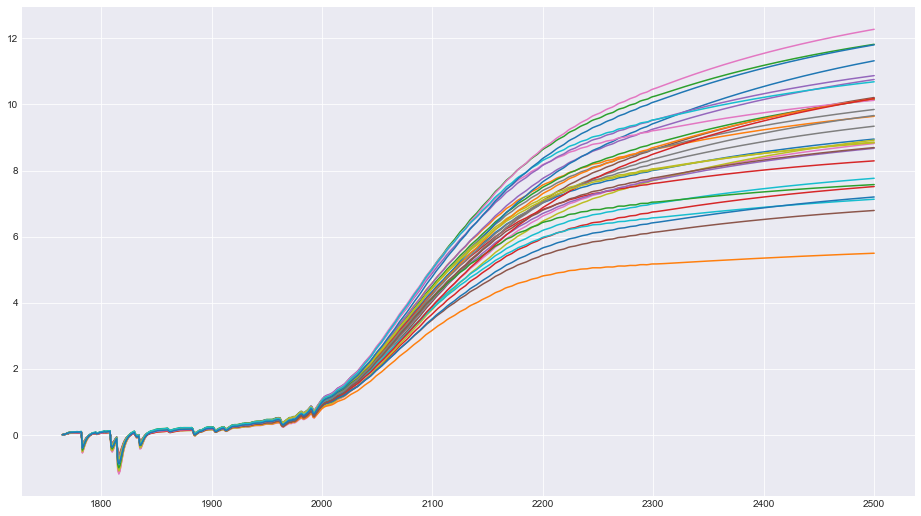Some, but not all, of the higher end scenarios have been constrained out, but there is still quite a large range of total temperature change projected for 2500 even under this constraint.

From these constraints it is possible to obtain posterior distributions on effective radiative forcing, ECS, TCR, TCRE and other metrics.

## Restart runs¶

Sometimes you may want to spin up FaIR in emissions-driven mode, and then impose a constant forcing or other scenario from a certain point in the future. The configuration of FaIR can be saved and re-used. This can be useful for investigating things like the climate change commitment from past emissions, because the carbon cycle and ocean thermal response will not be in their pre-industrial state.

Note the extra tuple element (`restart`) in the call to `fair_scm`. To activate, set the `restart_out = True` keyword in the first run, and `restart_in` keyword in the second run.

Currently, this is only possible when going from a CO2-only run to a CO2-only run, or a multi-forcing run to a CO2-only run. In this example we will run from 1765 to 2020 with all forcing agents, and then investigate the totally hypothetical scenario of a zeroing of CO2 emissions from 2020, with a constant non-CO2 radiative forcing.

```# Going from all-forcing to CO2 only. Note natural forcing turned off.
C1, F1, T1, restart = fair_scm(
emissions   = rcp45.Emissions.emissions[:255,:],
natural     = fair.ancil.natural.Emissions.emissions[:255,:],
restart_out = True,
F_solar     = 0.,
F_volcanic  = 0.
)

# constant non-CO2 forcing from 2019 to 2500
nonco2_rf = np.sum(F1[-1,1:]) * np.ones(481)

# run forward to 2100 with zero CO2 emissions and constant non-CO2 forcing
C2, F2, T2 = fair_scm(
emissions   = 0.,
other_rf    = nonco2_rf,
useMultigas = False,
restart_in  = restart
)

# do a normal RCP4.5 run for comparison
C45, F45, T45 = fair_scm(
emissions  = rcp45.Emissions.emissions,
F_solar    = 0.,
F_volcanic = 0.
)
```
```fig = plt.figure()

ax1.plot(rcp45.Emissions.year, np.concatenate((C1[:,0], C2)), color='blue',
label='zero CO2 emissions, constant non-CO2 forcing')
ax2.plot(rcp45.Emissions.year, np.concatenate((np.sum(F1,axis=1), F2)), color='blue',
label='Emissions driven')
ax3.plot(rcp45.Emissions.year, np.concatenate((T1, T2)), color='blue',
label='Emissions driven')

ax1.plot(rcp45.Emissions.year, C45[:,0], color='red', label='RCP4.5')
ax2.plot(rcp45.Emissions.year, np.sum(F45,axis=1), color='red', label='RCP4.5')
ax3.plot(rcp45.Emissions.year, T45, color='red', label='RCP4.5')

ax1.set_ylabel('CO2 concentrations, ppm')
ax3.set_ylabel('Temperature anomaly, K')

ax1.set_xlabel('year')
ax2.set_xlabel('year')
ax3.set_xlabel('year')
ax1.legend();
```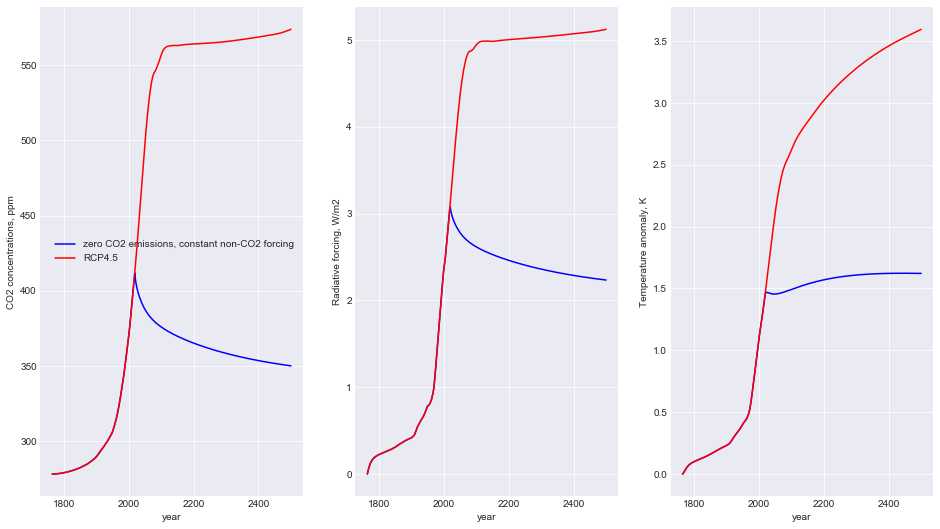## Switching the carbon cycle¶

The carbon cycle can be sped up by a factor of about 2 by using the Generalised Impulse Response (GIR) forumlation (Leach et al., 2020) by setting `gir_carbon_cycle` to `True`. This is likely to be the default in the future.

While there are some differences between the two methods for high temperature/carbon scenarios after 2200, the real-world (and full-complexity) model response at this level of emissions is so uncertain that this level of approximation is sufficient.

```C1,F1,T1 = fair_scm(emissions=rcp85.Emissions.emissions)
C2,F2,T2 = fair_scm(emissions=rcp85.Emissions.emissions, gir_carbon_cycle=True)
C3,F3,T3 = fair_scm(emissions=rcp26.Emissions.emissions)
C4,F4,T4 = fair_scm(emissions=rcp26.Emissions.emissions, gir_carbon_cycle=True)
fig =plt.figure()
ax1.plot(np.arange(1765,2501), C1[:,0], label='RCP8.5 FaIR v1');
ax1.plot(np.arange(1765,2501), C2[:,0], label='RCP8.5 GIR');
ax1.plot(np.arange(1765,2501), C3[:,0], label='RCP2.6 FaIR v1');
ax1.plot(np.arange(1765,2501), C4[:,0], label='RCP2.6 GIR');
ax1.legend()
ax1.set_title('CO2 concentrations')
ax1.set_ylabel('ppm')
ax2.plot(np.arange(1765,2501), T1);
ax2.plot(np.arange(1765,2501), T2);
ax2.plot(np.arange(1765,2501), T3);
ax2.plot(np.arange(1765,2501), T4);
ax2.set_title('Temperature')
ax2.set_ylabel('K');
```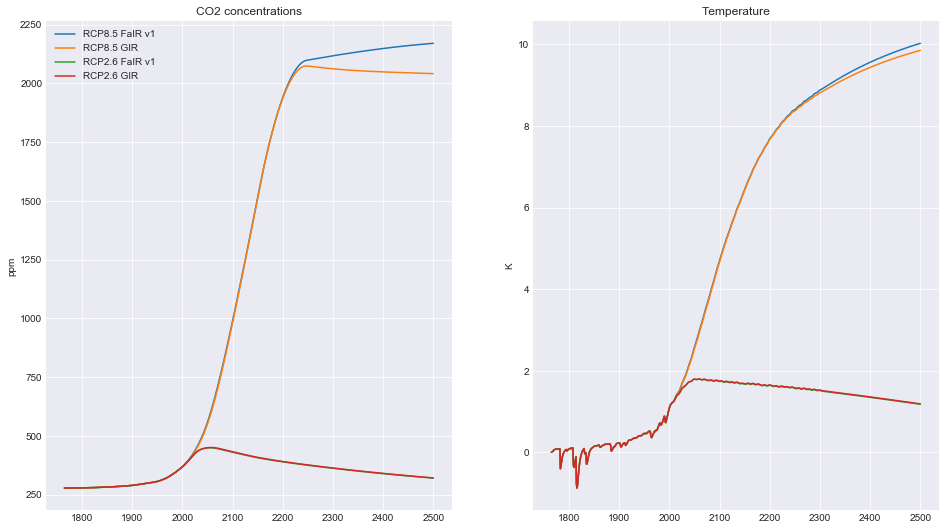## Geoffroy temperature function¶

We can use a different forcing to temperature coupling other than the one provided by default in FaIR. The two-layer model of Geoffroy et al., (2013 a, b) is mathematically equivalent but is specified in terms of ocean heat capacity, heat exchange and climate feedback, and allows for diagnosis of ocean heat uptake.

The call to `fair_scm` takes different parameters. Set `temperature_function="Geoffroy"` to use the two-layer model. `lambda_global` sets the climate feedback parameter, `ocean_heat_capacity` sets ocean heat capacity for the mixed and deep layers (2-element array), `deep_ocean_efficacy` is the efficacy factor and `ocean_heat_exchange` is the heat transfer coefficient between the two layers. It can be shown that the two specifications are mathematically equivalent - slight differences in the temperature response are probably due to timestepping and/or imprecision in the input parameters.

Below, the model response is tuned based on the MIROC5 model (see the Geoffroy papers for parameters).

Thanks to Zeb Nicholls and Glen Harris for implementations of the two-layer model, some of which was used here.

```fig = plt.figure()

C, F, T_irm = fair.forward.fair_scm(
emissions = rcp45.Emissions.emissions,
tcrecs=np.array([1.803,2.816]),
F2x=4.45,
scale_F2x=False,
d=np.array([337.4, 3.53]),
)

C, F, T_tlm, lambda_eff, ohc, heatflux = fair.forward.fair_scm(
emissions = rcp45.Emissions.emissions,
temperature_function='Geoffroy',
lambda_global=1.58,
ocean_heat_capacity=np.array([8.7,158]),
deep_ocean_efficacy=1.19,
ocean_heat_exchange=0.73,
F2x=4.45,
scale_F2x=False
)

ax1.plot(rcp45.Emissions.year, T_tlm, label='Geoffroy definition');
ax1.plot(rcp45.Emissions.year, T_irm, label='Impulse response definition')
ax1.legend()
ax1.set_title('Global mean surface temperature')
ax1.set_ylabel('K')
ax2.plot(rcp45.Emissions.year, ohc)
ax2.set_title('Ocean heat uptake')
ax2.set_ylabel('J')
ax3.plot(rcp45.Emissions.year, heatflux)
ax3.set_title('TOA energy imbalance')
ax3.set_ylabel('W m\$^{-2}\$')
ax4.plot(rcp45.Emissions.year, lambda_eff)
ax4.set_title('Effective climate feedback')
ax4.set_ylabel('W m^\${-2}\$ K\$^{-1}\$')
```
```Text(0, 0.5, 'W m^\${-2}\$ K\$^{-1}\$')
```